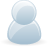# Support Forum

or## ACS758 current sensor for Windows 10 IOT C#

4223 Views - Created 03/06/2017

03/06/2017

Posted by:
CougarKenI am very fueint in C# code however I am having trouble with the ADC PI Plus and the ACS758 sensor.I had this working with an Aruino but have switched to a Raspberry Pi with Windows 10 IOT.The problem I have is that no matter what I do can only return a value of 2.310 plus or minus .010I have the same set up as your example with the ACS712 the only thing that is differant is the ACS758 is a 50 Amp sensor.It seems as if it is reading a differance between inputs but can not confirm that.The arduino code used the raw value then convert to mV then to amps.The sample code for windows 10 IOT just shows how to get the voltage and not the current but my problem is that the voltage reading is alwasy the same even when I apply a 30 Amp or 40 Amp load to thru the sensor.Any help would be great, Thenks

03/06/2017

Posted by:
andrewLocation:
United Kingdom

Hi

I haven't tested the acs758 sensor on the ADC Pi Plus but looking through the datasheet I couldn't see anything that would stop it from working with the board. Have you tried measuring the input on the ADC Pi Plus to see if that is showing 2.310 volts and if the sensor uses the same 5V supply as the Raspberry Pi?

Could you let me know the full part number for the sensor you are using so I can order one to test with an ADC Pi Plus?

03/06/2017

Posted by:
CougarKenThis is the one I have, https://www.amazon.com/Bi-direction-Current-Sensor-Module-Analog/dp/B00CEJ48KI/ref=sr_1_3/145-9708143-7840938?ie=UTF8&qid=1496522522&sr=8-3&keywords=ACS758+ModuleHere is a web page that shows the sensor and arduino code,http://henrysbench.capnfatz.com/henrys-bench/arduino-current-measurements/acs758-arduino-current-sensor-tutorial/To answer your question, yes the voltage is the same as when measured with a meter at the pin and yes, I am am powering the sensor from the 5V pin and ground on the ADC Plus just like it shows your example for the ACS712 project.

04/06/2017

Posted by:
CougarKenFYI,I have 3 different sensors all the same as listed above. I have tried all three with the same results.Just for the hell of it I hooked up the old Arduino to the circuit and it is reading the amperage correctly on all three sensors.This is the Arduino code it is C# as well.int mVperAmp = 40;int ACSoffset = 2500;int RawValue= 0;double Voltage = 0;double Amps = 0;RawValue = analogRead(analogIn);Voltage = (RawValue / 1023.0) * 5000; // Gets you mVAmps = ((Voltage - ACSoffset) / mVperAmp);The only difference is that with the Arduino I have to manually code the sample rate since there is no built in way of doing it. Without doing this the amp output would be Positive or Negative depending when it grabbed the reading since AC current is a sine wave as well as it may or may not be the actual amperage depending on where it was at in the wave. So the only difference is the Raspberry Pi and the ADC Pi Plus. If the Pi would have an analog input this may have been easier.Maybe it’s a Pi thing or an ADC Pi Plus thing I don’t know.

04/06/2017

Posted by:
andrewLocation:
United Kingdom

The problem could be that the sample rate of the ADC Pi Plus is too slow to measure the AC sinewave. The sample rate of the ADC in 18-bit mode is 3.75 samples per second so if you are measuring mains 60Hz AC voltage then the voltage would oscillate on either side of the centre voltage 16 times during a single sample which would be averaged out by the ADC to the centre voltage. I am guessing that the 5V power supply you are using is slightly below 5V and the voltage powering the sensor is around 4.62V which would explain the centre voltage from the sensor being 2.31V. With the ADC in 12-bit mode, you would still only get four samples per cycle on a 60Hz AC signal which would probably not be enough to get an accurate reading.

It may be possible to use an RMS-to-DC converter chip to change the AC voltage into a stable DC voltage that you could then read using the ADC Pi Plus. There are several manufacturers that make RMS-to-DC converters including Linear, Analog and Maxim. Depending on how the RMS-to-DC converter works you may need to find a way of offsetting the output from the sensor so it oscillates on either side of 0V instead of 2.31V.